Courses

# Computer Science And Information Technology - CS 2017 GATE Paper - 2 (Practice Test)

## 65 Questions MCQ Test GATE Computer Science Engineering(CSE) 2022 Mock Test Series | Computer Science And Information Technology - CS 2017 GATE Paper - 2 (Practice Test)

Description
This mock test of Computer Science And Information Technology - CS 2017 GATE Paper - 2 (Practice Test) for GATE helps you for every GATE entrance exam. This contains 65 Multiple Choice Questions for GATE Computer Science And Information Technology - CS 2017 GATE Paper - 2 (Practice Test) (mcq) to study with solutions a complete question bank. The solved questions answers in this Computer Science And Information Technology - CS 2017 GATE Paper - 2 (Practice Test) quiz give you a good mix of easy questions and tough questions. GATE students definitely take this Computer Science And Information Technology - CS 2017 GATE Paper - 2 (Practice Test) exercise for a better result in the exam. You can find other Computer Science And Information Technology - CS 2017 GATE Paper - 2 (Practice Test) extra questions, long questions & short questions for GATE on EduRev as well by searching above.
QUESTION: 1

### A test has questions worth 100 marks totals. There are two types of questions, multiple choice questions are worth 3 marks each and essay questions are worth 11 marks each. How many multiple choice questions does the exam have?

Solution:

Let us consider all choices :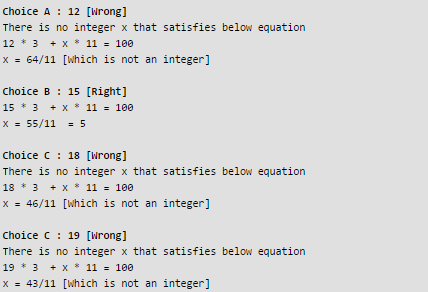QUESTION: 2

### There are 5 buildings called V, W, X, Y, Z in a row (not necessarily in order). V is to the West of W, Z is to the East of X and the West of V. W is to West of Y. Which building is in the middle?

Solution:

V is to the West of W,

V

W

Z is to the East of X and the West of V.

X

Z

V

W

W is to West of Y.

X

Z

V

W

Y

Therefore, V is in middle. So option A is correct.

QUESTION: 3

### Saturn is __________ to be seen on a clear night with the naked eye?

Solution:

With nouns, enough comes before noun.
In the given question, enough is used with bright which is an adjective, so enough will come after the adjective.
So bright enough is the correct option.

QUESTION: 4

There are 3 red socks, 4 green socks and 3 blue socks. You choose 2 socks. The probability that they are of the same color is

Solution:

Ways to collect both Red = 3C2

Ways to collect both Green = 3C2

Ways to collect both Blue = 4C2

Total Ways = 10C2

P(Socks of Same Colour) = (3C2 + 4C2+ 3C2 ) /  10C2 = 4/15

QUESTION: 5

Chose the option with words that are not synonyms.

Solution:
• Yield - produce or provide
• Resistance - the refusal to accept or comply with something. Rest are all synonyms. Hence, (D) is correct.
QUESTION: 6

The numbers of the roots of ex + 0.5x2 -2 = 0 in the range [-5, 5] are

Solution:

The given function is a continuous function in range [-5, 5]. The Intermediate Value Theorem states if a continuous function has values of opposite sign inside an interval, then it has a root in that interval. The function changes sign twice in range [-5, 5].

1. It becomes positive to negative in range [-5, 0] when ex + 0.5x2 becomes less than 2.
2. It becomes positive to negative in range [0, 5] when ex + 0.5x2 becomes more than 2.
QUESTION: 7

There are three boxes, one contains apples, another contains oranges and last one contains both apples and oranges. All three are known to be incorrectly labelled. You are permitted to open just one box and then pull out and inspect only one fruit. Which box would you open to determine the contents of all three boxes?

Solution:

In given question there are three boxes incorrectly labeled:

• Box 3 labeled as Apples and Oranges - Original Content can be Apple or Orangle (This box will have only one fruit, so whichever we pick would  be the correct label). Let's say the picked item was Apple, therefore the box labeled Orange will have Apples and Oranges.)
• Box 2 - Oranges - This box will definitely not have orange (as its mislabeled) and it can not contain only apple (As it is in box 3) - So this will have Original Content- both oranges and Apples
• Box 1 - Apples - This is the leftover box and it can now have Original Content - Orange

Therefore option B is correct

QUESTION: 8

X is 30 digit number starting with 4 followed 7. Then number X3 will have

Solution:

X = 4777....(till 30 digits)
or X = 4.7777⋯ ∗ 1029
X29 = (4.777⋯∗1029)3

= (4.777…)∗ 10 87
So, (X3 ) requires 3 + 87 = 90 digits.

QUESTION: 9

"We lived in culture and denied any merit to literally works, Considering them important only when they were handmaidens to something seemingly more urgent - namely ideology. This was a country where all gestures even the most private , interpreted as political terms." The author's believes that ideology is not as important as literature is revealed by the word :

Solution:
QUESTION: 10

An air pressure contour line join the locations in the region having the same atmospheric pressure. The following is the air pressure contour of a geographical region. The contour line are shown as 0.05 bar intervals in this plot.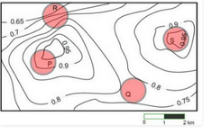Q. If the probability of thunderstorm is given by how fast air pressure rises or drops over a region. Which of the following region is most likely to have thunderstorm.

Solution:

Region R has maximum air pressure variation as it  which has maximum number of lines crossing the area. Therefore option C is correct

Alternate Solution
We have to see for the region which has maximum change in air pressure for thunderstorm.
In region P – the air pressure changes only once by 0.05 as only 2 lines are crossing the region. The first line with the air pressure 0.95 and the next one is with pressure 0.9.
In region Q – No line crosses the region which implies that the air pressure doesn’t change.
In region R – the air pressure changes at 4 places resulting in maximum change in air pressure in the whole region. The minimum pressure in R is 0.65 and the maximum is 0.8.
In region S – the air pressure in the region in 0.95 and other contouring line is outside the region thus no change in air pressure within the region.
Thus the probability of thunderstorm is maximum in region R.

QUESTION: 11

Let pqr denote the statement "It is raining", "It is cold", and "It is pleasant", respectively. Then the statement "It is not raining and it is pleasant, and it is not pleasant only if it is raining and it is cold" is represented by:

Solution:
QUESTION: 12

Match the following according to input(from the left column) to the compiler phase(in the right column) that process it: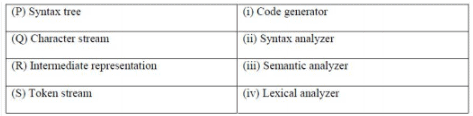Solution:

P - iii Syntax tree is input to semantic analyser Q - iv Character stream is input to lexical analyser. R - i Intermediate code is input to code generator S -ii  Token stream is input to syntax analyser. Therefore option C

QUESTION: 13

In a file allocation system, which of the following allocation scheme(s) can be used if no external fragmentation is allowed?

I. Contiguous
III. Indexed

Solution:

In contiguous allocation there is always possibility of external fragmentation. Both indexed and linked allocation are free from external fragmentation.

Therefore, option D

QUESTION: 14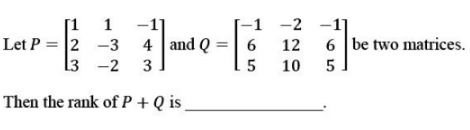Note: This question appeared as Numerical Answer Question in GATE.

Solution: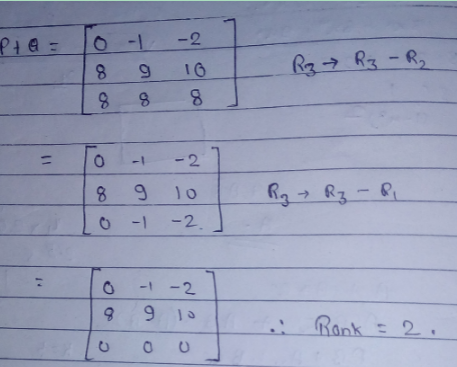QUESTION: 15

The representation of the value of a 16-bit unsigned integer X in a hexadecimal number system is BCA9. The representation of the value of X in octal number system is:

Solution: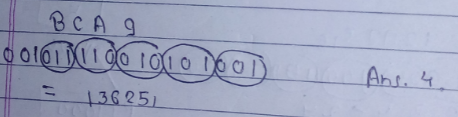QUESTION: 16

An ER model of a database consists of entity types A and B. These are connected by a relationship R which does not have its own attribute. Under which of the following conditions, can the relational table for R be merged with that of A?

Solution:

In one to many or many to one participation, relation is merged in table which is on many side and if there is total participation then relation is merged in that side. Therefore, option C is correct

QUESTION: 17

Match the algorithms with their time complexities: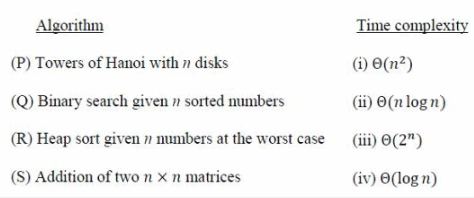Solution:
• Tower of Hanoi - Ɵ(2n)
• Heap sort worst case - Ɵ(n log n)
• Binary Search - Ɵ(log n)
• Addition of two nxn matrices - Ɵ (n2)

Therefore Option C is correct

QUESTION: 18

Consider the following statements about the routing protocols, Routing Information Protocol(RIP) and Open Shortest Path First(OSPF) in an IPv4 network.

I. RIP uses distance vector routing
II. RIP packets are sent using UDP
III. OSPF packets are sent using TCP
IV. OSPF operation is based on link-state routing
Which of the following above are CORRECT?

Solution:
QUESTION: 19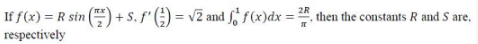Solution:QUESTION: 20

The maximum number of IPv4 router address addresses that can be listed in the record route (RR) option field of an IPv4 header is ____.
Note: This question appeared as Numerical Answer Type.

Solution:

Record Route is an optional field used to record address.
In IPv4 header, 40 bytes are reserved for OPTIONS. For Record Route to stores, 1 byte is used to store type of option, 1 byte for length and 1 byte for pointer. Out of 40, 37 bytes are left.
An IP4 address takes 32 bits or 4 bytes.
We can store at most floor(37/4) = 9 router addresses.

Alternate Solution
In IPV4 frame format there are 40 bytes of option field, out of which only 38 bytes can be used, 2 bytes are reserved . Also one IPV4 adress is of 4 bytes
Thus maxmimum IPV4 address that can be hold <= 38/4 = 9

QUESTION: 21

Consider the following tables T1 and T2: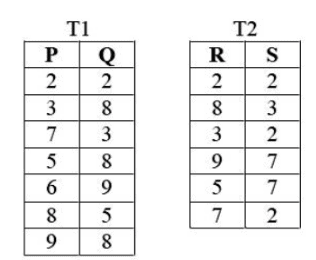Q. In table T1, P is the primary key, Q is the foreign key referencing R in table T2 with on-delete cascade and on-update cascade. In table T2, R is the primary key and S is the foreign key referencing P in the table T1 with on-delete set NULL and on-update cascade. In order to delete record (3,8) from table, numbers of additional record that need to be deleted from table T1 is

Solution:

Given,

• Q -> R(Primary Key)
• S -> P (Primary Key)

Entry to be deleted - P (3) and Q(8)

• Q can be deleted directly
• Now, S - > P but the relationship given is on delete set NULL, Therefore when  we delete 3 from  T1 ,the  entry in T2 having 3 will be NULL.

Therfore, Option A  - Answer is 0 entries

QUESTION: 22

Breath First Search(BFS) has been implemented using queue data structure.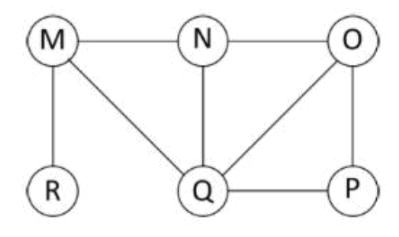Q. Which one of the following is a possible order of visiting the nodes in the graph above.

Solution:

In BFS, we print a starting node, then its adjacent, then adjacent of adjacent, and so on..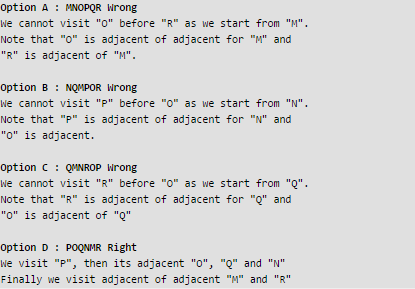QUESTION: 23

Consider a socket API on Linux machine that supports UDP socket. A connected UDP socket is a UDP socket on which connect function has already been called. Which of the following statements is/are correct ?

I. A connected UDP socket can be used to communicate with multiple peers simultaneously.

II. A process can successfully call connect function again for an already connected UDP socket.

Solution:

I. A connected UDP socket can be used to communicate with multiple peers simultaneously is WRONG : Even if UDP is connectionless, every UDP socket has a single specified IP number and port number to connect to. Therefore we can not use same socket to connect to multiple peers simultaneously. II. A process can successfully call connect function again for an already connected UDP socket is RIGHT : We can call connect again to connect to a new peer and disconnect previous peer if implementation allows

QUESTION: 24

Let L1 and L2 be any context-free language and R be any regular language. Then, which of the following is correct ?

I. L1 ∪ L2 is context-free.
II. L1' is context-free.
III. L1-R is context-free.
IV. L1 ∩ L2

Solution:

Context free language are closed under union and difference with regular language.
It is not closed under complementation and intersection . Complement of CFL is recursive language .

QUESTION: 25

Match the following: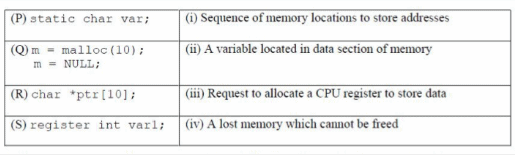Solution:
• static char var; -> A variable located in data section of memory as it is static in nature
• m = malloc(10); m = null; ->This is a lost memory which cannot be freed as m=NULL
• Char *Ptr; -> 10 memory locations of char type are allocated to store addresses
• register int var1;-> Request to allocate a CPU register to store data
QUESTION: 26

Identity the language generated by following grammar where S is the start variable.

S --> XY
X --> aX | a
Y --> aYb | ∈

Solution:

S --> XY
X --> aX | a // This produces only "a"
Y --> aYb | ∈ // This produces and "a" for every "b"

Option (A) and (B) are wrong because n can be zero also due to epsilon in Y Option (D) is wrong because Y-->aYb produces equal number of a's and b's.
Since there is one variable X which produces at least one a.
Therefore numbers of a's are always greater than numbers of b's.

QUESTION: 27

G is undirected graph with n vertices and 25 edges such that each vertex has degree at least 3. Then the maximum possible value of n is ________

Solution:

As per handshaking lemma,

Sum of degree of all vertices < = 2 * no. of edges.

Let v be number of vertices in graph.
==> 3*v <= 2*25
==> Minimum value of v = 16

QUESTION: 28

The minimum possible number of states of a deterministic finite automaton that accepts a regular language L = {w1aw2 | w1, w2 ∈{a,b}* , |w1| = 2, w2>=3} is_______

Solution: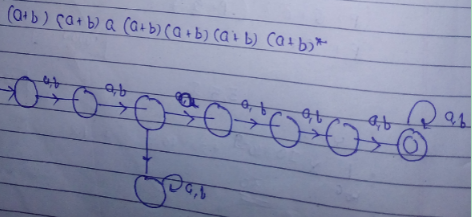QUESTION: 29

Given the following binary number in 32 bit (single precision) IEEE-754 format:

00111110011011010000000000000000

Q. The decimal value closest to this floating-point number is:

Solution:

In 32-bit IEEE-754 format

1st bit represent sign
2-9th bit represent exponent and 10-32 represent Mantissa (Fraction part)

Sign = 0, so positive
2-9 bits — 01111100 when subtracted by 01111111 i.e., 126 decimal value gives -> 0000 0011
Which is -3.(negative as the value is less than 126)
As number is less than 126 it is subtracted otherwise 126 would have been subtracted from it in 32 bit representation.
Mantissa is normal ,hence, 1.M can be used .Which is 1.1101101.
Thus,
Data + 1.1101101 * 2^-3 (±M * B^(±e))
Mantissa shift right 3 times ->
+0.0011101101
= 0.228
= 2.28 * 10^-1
Thus, option c is correct.

QUESTION: 30

A Circular queue has been implemented using singly linked list where each node consists of a value and a pointer to next node. We maintain exactly two pointers FRONT and REAR pointing to the front node and rear node of queue. Which of the following statements is/are correct for circular queue so that insertion and deletion operations can be performed in O(1) i.e. constant time.

I. Next pointer of front node points to the rear node.

II. Next pointer of rear node points to the front node.

Solution:
QUESTION: 31

Which of the following statements about the parser is/are correct?

I. Canonical LR is more powerful than SLR.

II. SLR is more powerful than LALR.

III. SLR is more powerful than canonical LR.

Solution:

LR parsers in term of power CLR > LALR > SLR > LR(0) Therefore, Option A is only correct option

QUESTION: 32

Consider a quadratic equation x2 - 13x + 36 = 0 with coefficients in a base b. The solutions of this equation in the same base b are x = 5 and x = 6. Then b = ______.

Note: This question appeared as Numerical Answer Type in GATE.

Solution:

x2 – 13x + 36 = 0
==> x2 – (b + 3)x + (3*b + 6) = 0 …..
{ expanding numbers in base b )
putting the values of x we get b = 8.

QUESTION: 33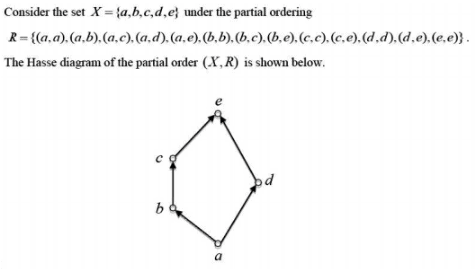Q. The minimum number of ordered pairs that need to be added to R to make (X, R) a lattice is _____.
Note: This question appeared as Numerical Answer Type.

Solution: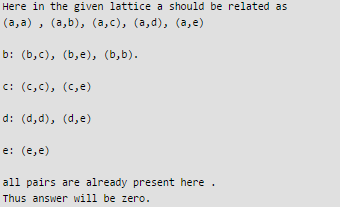QUESTION: 34

Which of the following is/are shared by all the threads in a process?

I. Program Counter
II. Stack
IV. Registers

Solution:

Every thread have its own stack , register, and PC, so only address space that is shared by all thread for a single process. Therefore,  option B is correct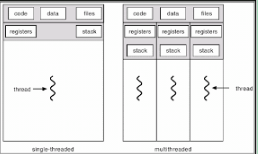QUESTION: 35

Consider the following function implemented in C: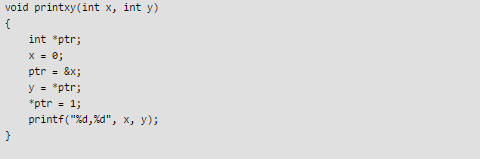Q. The output of the printxy(1,1) is

Solution: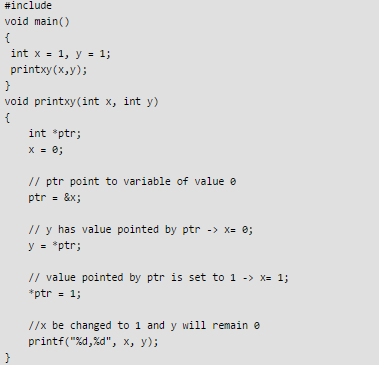QUESTION: 36

A system shares 9 tape drives. The current allocation and maximum requirement of tape drives for 3 processes are shown below: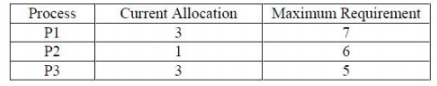Q. Which of the following best describes the current state of the system?

Solution: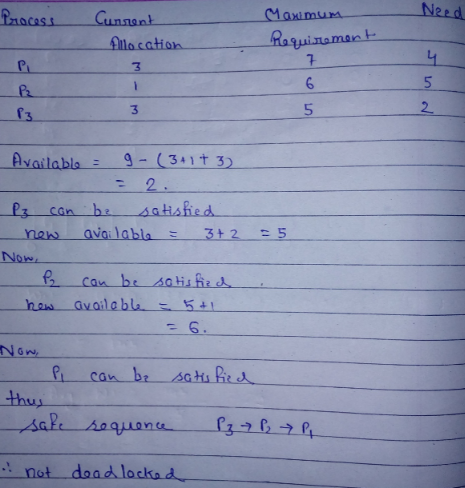QUESTION: 37

The read access times and the hit ratios for different caches in a memory hierarchy are as given below: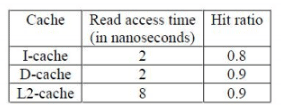Q. The read access time of main memory in 90 nanoseconds. Assume that the caches use the referred-word-first read policy and the writeback policy. Assume that all the caches are direct mapped caches. Assume that the dirty bit is always 0 for all the blocks in the caches. In execution of a program, 60% od memory reads are for instruction fetch and 40% are for memory operand fetch. The average read access time in nanoseconds (up to 2 decimal places) is _________

Solution:
QUESTION: 38

Consider the C program fragment below which is meant to divide x by y using repeated subtractions. The variable x, y, q and r are all unsigned int.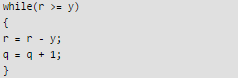Q. Which of the following conditions on the variables x, y, q and r before the execution of the fragment will ensure that the loop terminates in a state satisfying the condition x == (y*q + r)?

Solution:

x == (y * q + r) x= product, y= multiplicand, q = quotient , r =  remainder

• For loop to be terminated, the quotient has to be 0, so only options C and D are left
• If q=0 -> r=x should apply

Therefore, option C is most appropriate

QUESTION: 39

Let δ denote the transition function and α denoted the extended transition function of the ε-NFA whose transition table is given below: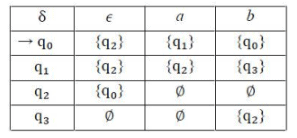Q. Then, α (q2,aba) is

Solution:

Extended transition function describes what happens when we start in any state and follow any sequence of inputs.
Since this is an epsilon NFA so we have to consider the epsilon moves also and see which states we can reach after the input string is over.
The starting state is q2, from q2 transition with input a is dead so we have to look for epsilon transition. With epsilon transition we reach to q0, at q0 we have a transition with input symbol a so we reach to state q1.
From q1 we can take transition with symbol b and reach state q3 but from q3 we have no further transition with symbol a as input so we have to take another transition from state q1 that is the epsilon transition which goes to state q2.
From q2 we reach to state q0 and read input b and then read input a and reach state q1. So q1 is one of the state of extended transition function.
From q1 we can reach q2 with epsilon as input (mans with no input) and from q2 we can reach q0 with epsilon move so state q2 and q0 are also part of extended transition function.
So state q1, q2, q0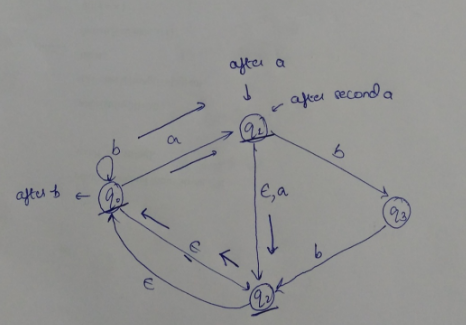QUESTION: 40

Consider the following expression grammar G.

E -> E - T | T
T -> T + F | F
F -> (E) | id

Q. Which of the following grammars are not left recursive, but equivalent to G.

Solution:

We know for left recursion : A -> Aα/β
After removing left recursion it can be written as A->βA’
A’->αA’/ε
Thus for : E->E- T/T
α= -T , β= T . thus new production after removing left recursion
is E->TE’ and E’->- TE’/ ε
T->FT’ and T’->+FT’/ ε
F->(E)/id.

QUESTION: 41

In a two-level cache system, the access times of L1 and L2 1 and 8 clock cycles, respectively. The miss penalty from the L2 cache to main memory is 18 clock cycles. The miss rate of L1 cache is twice that of L2. The average memory access time(AMAT) of this cache system is 2 cycles. The miss rates of L1 and L2 respectively are:

Solution:
• Access time of L1 = 1
• Access Time of L2=8
• miss penalty  L1 cache (2*L2)  = 18*2 = 2*a
• miss penalty  L2 cache say a = 18
• AMAT (average memory access time) =2

AMAT = Access time of L1 + (MissRate L1 * miss penalty  L1)  where miss penalty L1 = Access time of L2 + (MissRate L2 * miss penalty L2) 2 = 1+ 2*a *(8 + a* 18) Solving the equation, a=0.111

QUESTION: 42

Consider the following C program: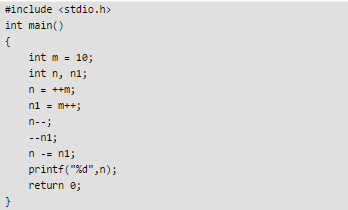Q. The output of the program is ______.

Note: This questions appeared as Numerical Answer Type.

Solution: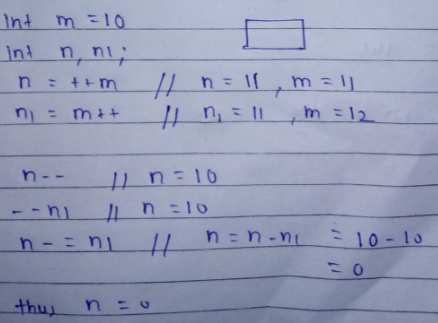QUESTION: 43

Consider two hosts X and Y, connected by a single direct link of rate 106 bits/sec. The distance between the two hosts is 10,000 km and the propagation speed along the link is 2 x 108 m/s. Hosts X send a file of 50,000 bytes as one large message to hosts Y continuously. Let the transmission and propagation delays be p milliseconds and q milliseconds, respectively. Then the vales of p and q are:

Solution:

Propagation delay = link length/ signal speed = (10^7)/(2*10^8)sec = 5 =.005 sec =50 msec Transmission delay = data length/signal bandwith = 50000*8/10^6 sec =.04 sec =400 msec

QUESTION: 44

If w, x, y, z are boolean variables, then which of the following in INCORRECT?

Solution: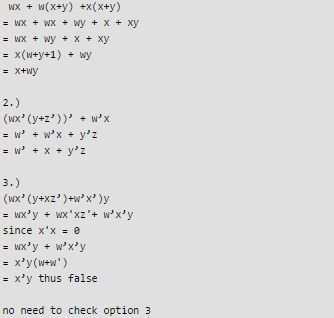QUESTION: 45

The pre-order traversal of a binary search tree is given by 12, 8, 6, 2, 7, 9, 10, 16, 15, 19, 17, 20. Then the post-order traversal of this tree is:

Solution: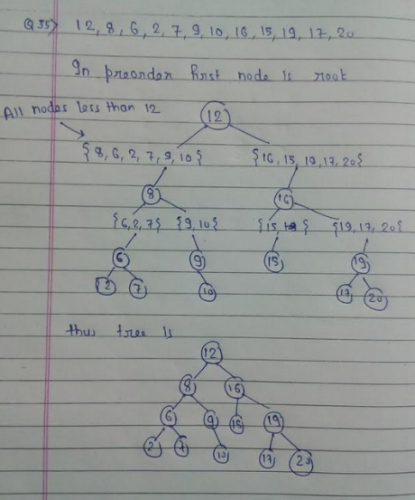QUESTION: 46

Consider the set of processes with arrival time(in milliseconds), CPU burst time (in milliseconds), and priority(0 is the highest priority) shown below. None of the processes have I/O burst time.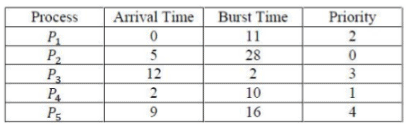Q. The average waiting time (in milliseconds) of all the processes using preemptive priority scheduling algorithm is ____.
Note: This question appeared as Numerical Answer Type.

Solution: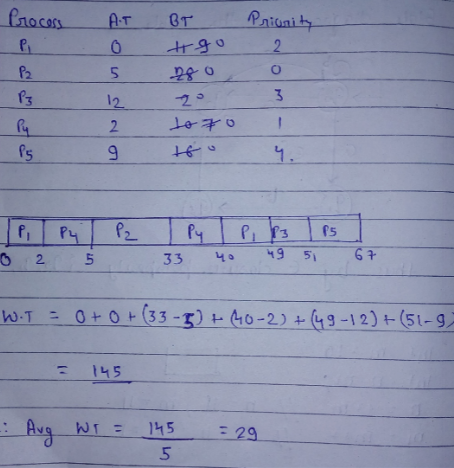QUESTION: 47

Consider the following snippet of a C program. Assume that swap(&x, &y) exchanges the contents of x and y.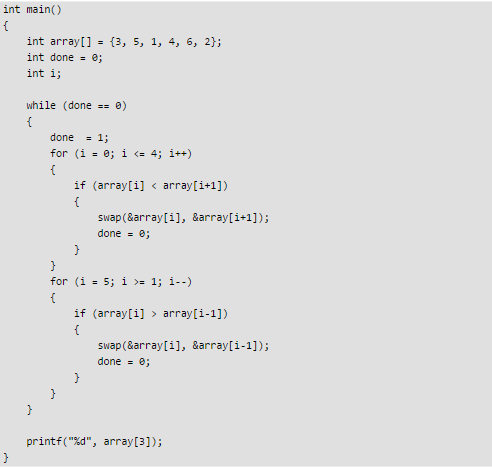Q. The output of the program is _____.

Note: This question appeared as Numerical Answer Type.

Solution:

After the execution of the for loop, the content of array: First time: 5 3 4 6 2 1 Second time: 6 5 4 3 2 1 Third time: 6 5 4 3 2 1 Now, when while loop execute again, done = 1 and first and second for loop if condition will not be satisfied . Therefore, At the end, value of arr = 3, Option C is correct. See this to understand more: [sourcecode language="CPP"] #include void swap(int *t, int *x) { int m; m = *t; *t = *x; *x = m; } int main() { int array[] = {3, 5, 1, 4, 6, 2}; int done = 0; int i; while (done == 0) { done = 1; for( i = 0; i <= 4; i++) { if(array[i] < array[i+1]) { swap(&array[i], &array[i+1]); done = 0; } } for (i = 5; i >= 1; i--) { if( array[i] > array[i-1]) { swap(&array[i], &array[i-1]); done = 0; } } } printf("%d", array); } [/sourcecode]

QUESTION: 48

Consider the following languages.
L1 = {ap | p is a prime number}
L2 = {anbmc2m | n >= 0, m >= 0}
L3 = {anbnc2n | n >= 0}
L4 = {anbn | n >= 1}

Q. Which of the following are CORRECT ?

I. L1 is context free but not regular.
II. L2 is not context free.
III. L3 is not context free but recursive
IV. L4 is deterministic context free

Solution: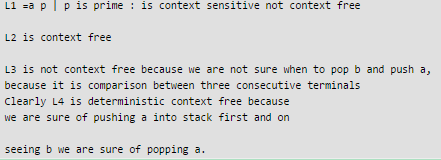QUESTION: 49

Consider the following C function.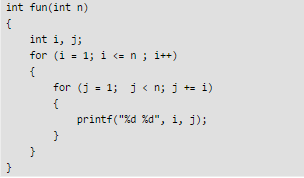Q. Time complexity of fun in terms of θ notation is:

Solution:

Let us see how many times the innermost statement "printf("%d %d", i, j);" is executed. For i = 1, the statement runs n times
The i'th iteration, statement runs Θ(n/i) times. Summing all iterations for i = 1 to n, we get Thus T(n) = Θ(n(1 + 1/2 +1/3 + ….)) = Θ(n log n). Note that the value of 1/1 + 1/2 + 1/3 + .... 1/n is approximately same as Log n for large values of n.

QUESTION: 50

Let L(R) be the language represented by regular expression R. Let L(G) be the language generated by a context free grammar G. Let L(M) be the language accepted by a Turing machine M. Which of the following decision problems are undecidable? I. Given a regular expression R and a string w, is w∈L(R)? II. Given a context-free grammar G, is L(G)=∅ III. Given a context-free grammar G, is L(G)=Σ for some alphabet Σ? IV. Given a Turing machine M and a string w, is w ∈ L(M)?

Solution:
1. Membership problem of regular language ->Decidable
2. Emptyness of CFL ->Decidable
3. L(G)=Σ problem of CFL  -> UNdecidable
4. Membership problem of RE language -> UNdecidable

Therefore , option D

QUESTION: 51

P and Q are considering to apply for job. The probability that p applies for job is 1/4. The probability that P applies for job given that Q applies for the job 1/2 and The probability that Q applies for job given that P applies for the job 1/3.The probability that P does not apply for job given that Q does not apply for the job .

Solution: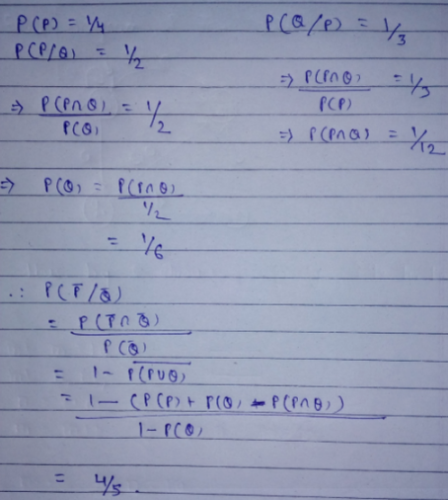QUESTION: 52

If the ordinary generating function of a sequence is: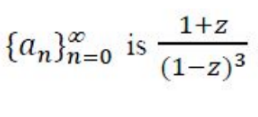then a3-a0 is equal to:

Solution:
QUESTION: 53

Consider the following program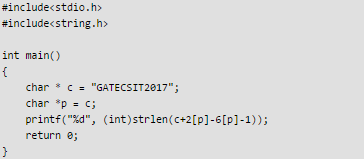Q. The Output of the following program is____

Solution: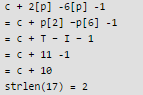QUESTION: 54

Consider a machine with byte addressable memory of 232 bytes divided into blocks of size 32 bytes. Assume a direct mapped cache having 512 cache lines is used with this machine. The size of tag field in bits is _____

Solution:

Total address space = 32 bit
Block size (B) = 5 bit
No. of bit to represent line no (L) = 9.
Tag bit + B + L = 32
==> X + 5 + 9 = 32
==> X = 18

QUESTION: 55

Consider a binary code that consists only four valid codewords as given below.
00000, 01011, 10101, 11110
Let minimum Hamming distance of code be p and maximum number of erroneous bits that can be corrected by the code be q. The value of p and q are:

Solution:

We need to find minimum hamming distance(difference in their corresponding bit position)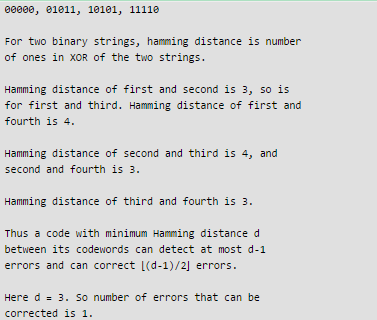QUESTION: 56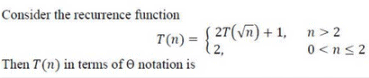Solution: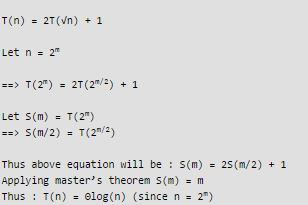QUESTION: 57

The next state table of a 2 bit saturating up-counter is given below.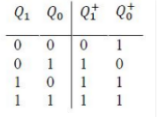Q. The counter is built as synchronous sequential circuit using T flip-flops. The value for T1 and T0 are

Solution: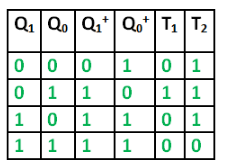Using above excitation table, T1 = Q'1Q0 = 0100 T0 = Q'1 + Q'0 = 1110 Therefore Option B

Another Solution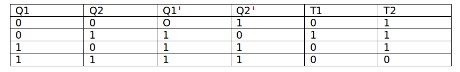T1 and T2 are filled by using the property that output of T FF will change when T=1 and will not change when T=0
Thus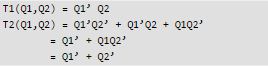QUESTION: 58

A message is made up entirely of characters from the set X = {P,Q,R,S,T} . The table of probabilities of each character is shown below :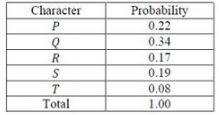Q. A message of 100 characters over X is encoded using Huffman coding. Then the excepted length of the encoded message in bits is _____

Solution: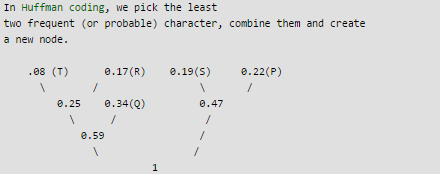Looking at above tree structure, Number of bits required by each: P - 2 Q - 2 R - 3 S - 2 T - 3 Therefore, excepted length of the encoded message = 3*0.8 + 3*0.17 +  2*0.19  + 2 *0.22 + 2*0.34 = 2.25 For 100 characters, 2.25*100 = 225 Therefore, option A is correct.

QUESTION: 59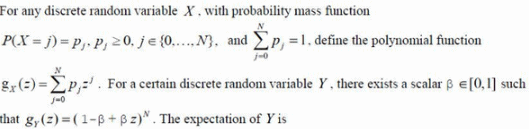Solution:

gy(z) = ((1- β) + βz)N Expanding  gy(z) we'll get binomial distribution  with n=N and p = β Mean of binomial distribution is E[Y] = n*p = Nβ which seems to be the only correct option here. Therefore, option B

QUESTION: 60

In a B+ tree, if the search-key value is 8 bytes long, the block size is 512 bytes and the block pointer is 2 bytes, then the maximum order of the B+ tree is ____.

Note: This question appeared as Numerical Answer Type.

Solution: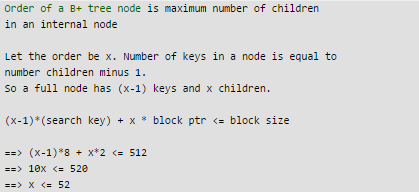QUESTION: 61

Consider the following database table named top_scorer.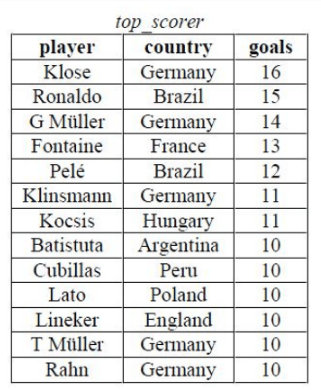Consider the following SQL query: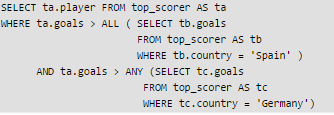Q. The number of tuples returned by the above SQL query is ____.

Note: This questions appeared as Numerical Answer Type.

Solution:

The query says we need to

1. Condition 1: Select players which have goals greater than ALL players of spain - This conditon will always be true as ALL (empty) always returns TRUE. AND
2. Condition 2: Any  player of Germany having 10 goals, so all the rows which are greater than 10 Goals will be returned.

Looking at the table, first 7 rows satisfy both the conditions.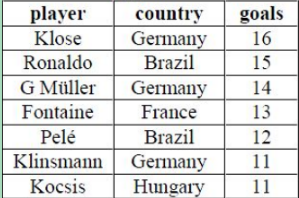Therefore, option B is true.

QUESTION: 62

If a random variable X has a Poisson distribution with mean 5, then the expression E[(X + 2)2] equals _____.

Note: This question appeared as Numerical Answer Type.

Solution: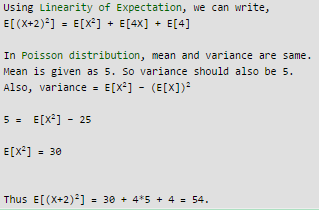QUESTION: 63

Given f(w, x, y, z) = Σm(0,1,2,3,7,8,10) + Σd(5,6,11,15), where d represents the don't-care condition in Karnaugh maps. Which of the following is a minimum product-of-sums(POS) form of f(w,x,y,z)?

Solution: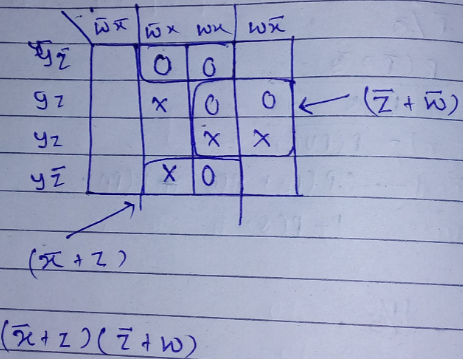QUESTION: 64

Two transactions T1 and T2 are given as:

T1: r1(X)w1(X)r1(Y)w1(Y) T2 : r2(Y)w2(Y)r2(Z)w2(Z)

where ri(V) denotes a read operation by transaction Ti on a variable V and wi(V) denotes a write operation by transaction Ti on a variable V. The total number of conflict serializable schedules that can be formed by T1 and T2 is ______

Note: This question appeared as Numerical Answer Type.

Solution:

For schedules to be conflict serializable they should be free from RW, WW, WR conflicts.
In the conflict serializable schedule we have to see that the operations in both the transactions occurring on same data item should not conflict. Data item y is shared between the two transactions so the read and write operations in T1 on data item y can produce RW, WR, WW conflict with the read and write operations of transaction T2 on data item y.
Another constraint is that the order of operations of each transaction should be maintained. We can not change the order of operation on transaction. Suppose if read(x) is before write(x) in T1 then it should be in the same order in resulting conflict serializable schedule.
In T1 we have two conflicting operations r1(y) and w1(y)
In T2 we have two conflicting operations r2(y) and w2(y)
Both the read and write of T1 on y should be performed together either before the read write pair of T2 or after read write pair to T2 because interleaving them will result in inconsistency because both these transaction are performing operation on same object.
There is only one way to have (conflict) serializable schedule as T1->T2, because last operation of T1 and first operation of T2 conflicts each other.
Now See How many schedules are conflict serializable to T2->T1.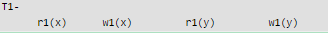Now See T2 from right, if we see T2 from right, see the first conflicting operation w2(z) and r2(z) don’t have any conflict with any operation, but w(y) has conflict Pick W2(y) and see, at how many places it can be there.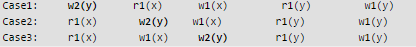Pick each case and see,how many positions other operation of T2 can take.
Case1:     w2(y)      r1(x)      w1(x)         r1(y)         w1(y)    How many positions w2(z) and r2(z) can take ? (note that these w2(z) and r2(z) cant come before w2(y)) that is 5C1 + 5C2 = 15 (either both can take same space or two different spaces) Now see, for each of these 15 positions, how many can r2(y) take ? Obiviously r2(y) cant come before w2(y) therefore one position. 15x1 = 15 total possible schedules from case 1.

Case2:     r1(x)       w1(y)    w1(x)         r1(y)         w1(y)    How many positions w2(z) and r2(z) can take ? that is 4C1 + 4C2 = 10 (either both can take same space or two different spaces) Now see, for each of these 10 positions, how many can r2(y) take ? Only 2 positions, because it has to come before w1(y). 10x2 = 20 total possible schedules from case 2.

Case3:     r1(x)       w1(x)     w2(y)        r1(y)         w1(y)    How many positions w2(z) and r2(x) can take ? that is 3C1 + 3C2 = 6 Now see, for each of these 6 positions, how many can r2(y) take ? Only 3 positions, because it has to come before w2(y). 6x3 = 18 total possible schedules from case 3. total schedules that are conflict serializable as T2->T1 = 15+20+18 = 53. total schedules that are conflict serializable as T1->T2 = 1.   total schedules that are conflict serializable as either T2->​​​​​​​T1  or  T1->T2 = 53+1 = 54.

QUESTION: 65

If the characteristic polynomial of a 3 × 3 matrix M over R (the set of real numbers) is λ3 - 4λ2 + aλ + 30, where a ∈ R, and one eigen value of M is 2, then the largest among the absolute values of the eigenvalues of M is _______
Note: This question appeared as Numerical Answer Type.

Solution:

λ3 - 4λ2 + aλ + 30 = 0
Since 2 is root . putting in eqn we get a = -11
λ3 - 4λ2 - 11λ + 30 =0
solving we get λ = 2, -3, 5.